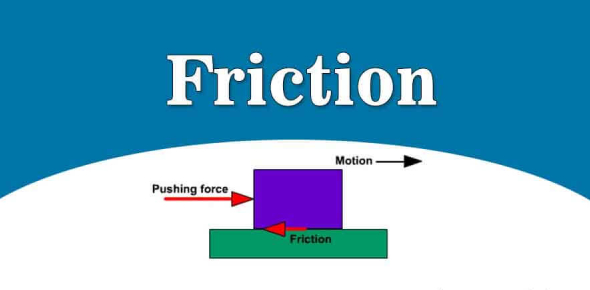# Basic Questions On Friction! Trivia Quiz

18 Questions | Attempts: 7048
ShareSettingsFriction is the force opposing the relative motion of solid surfaces, fluid layers, and material elements coming against each other. This quiz features questions concerning friction. You should be aware of what type of force is friction, which direction does friction act, is there friction when two objects are not in contact, is static friction larger than dynamic friction, and when friction is most experienced. Don’t let this quiz rub you the wrong way; you will ACE it.

• 1.
Friction is a .......... force.
• 2.
Which direction does Friction act?
• A.

Same

• B.

Opposite

• 3.
Gravity is a ........
• 4.
The object loses ......... as it falls.
• 5.
If an object experiences a frictional force the object loses ..........
• 6.
The displacement of the block is parallel to the surface of the ramp, therefore the frictional force is also parallel to the ramp but in the same direction of the displacement.
• A.

True

• B.

False

• 7.
There is friction when two objects are not in contact.
• A.

True

• B.

False

• 8.
Static friction is always larger than dynamic friction.
• A.

True

• B.

False

• 9.
Static friction and dynamic friction have same magnitudes.
• A.

True

• B.

False

• 10.
Can the energy that is lost flow back into the object?
• A.

Yes

• B.

No

• 11.
Friction is most often experienced when
• A.

When two objects are in contact

• B.

When two objects are not in contact

• 12.
kinetic energy is
• A.

Heat

• B.

Sound

• C.

Both

• 13.
Friction can be explained as.
• A.

Two rough surfaces rubbing together

• B.

Two smooth surfaces rubbing together

• C.

Two smooth surfaces apart from each other

• 14.
Dynamic friction is
• A.

Kinetic

• B.

Static

• 15.
When an objects starts to move, friction is
• A.

Increased

• B.

Decreased

• C.

Does not change

• 16.
If you push on a heavy object with a small force it will not slide. Friction will prevent it from sliding.
• A.

True

• B.

False

• 17.
Friction is caused by
• A.

Irregularities in an object

• B.

Regularities in an obect

• 18.
Static energy is
• A.

two solid objects that are moving relative to each other

• B.

two solid objects that are not moving relative to each other

## Related TopicsBack to top
×

Wait!
Here's an interesting quiz for you.